# Uncertain Multiobjective Programming and Uncertain Goal Programming

## Abstract

Multiobjective programming, known as multi-criteria or multi-attribute optimization, is the process of simultaneously optimizing two or more conflicting objectives. This paper aims to provide a new multiobjective programming named uncertain multiobjective programming that is a type of multiobjective programming involving uncertain variables. Some mathematical properties are also explored. Besides, uncertain goal programming is introduced as a compromise method for solving the uncertain multiobjective programming models. Both the uncertain multiobjective programming model and the uncertain goal programming model are transformed to crisp programming models with the help of the operational law of uncertain variables via inverse uncertainty distributions.

## Introduction

Multiobjective programming was introduced by Neumann and Morgenstern  to optimize two or more conflicting objectives subject to certain constraints. Then, Koopmans  introduced the concept of Pareto optimal solutions for the multiobjective programming problem. As a compromise method for solving multiobjective programming, goal programming was proposed by Charnes and Cooper . These models are all deterministic multiobjective programming models. However, the decision environment is usually full of indeterminacy. Stochastic programming was first studied by Dantzig  in 1955. Probability theory was introduced to multiobjective programming by Charnes and Cooper  and further developed by many researchers.

As we know, when we want to apply probability theory, adequate historical data are required to estimate the probability distribution. However, in many cases, there are no samples available to estimate the probability distribution. Then some domain experts are invited to evaluate the degree of belief that each event may happen. Kahneman and Tversky  pointed out that human beings usually overweight unlikely events. On the other hand, Liu  demonstrated that human beings usually estimate a much wider range of values than the object actually takes. This conservatism of human beings makes the belief degrees deviate far from the frequency . In order to deal with degree of belief, uncertainty theory was founded by Liu  in 2007 and perfected by Liu  in 2009. After born, uncertainty theory has also been widely applied to many other fields. The study of uncertain statistics was started by Liu  in 2010. Besides, Liu  introduced the principle of least squares method for uncertain statistics. Furthermore, Wang and Peng  proposed the method of moments. Uncertain risk analysis and uncertain reliability analysis were discussed by Liu . Uncertain set theory was proposed by Liu  and was also applied to inference control systems by Liu . Gao  successfully controlled an inverted pendulum using the uncertain inference rules. Peng and Chen  proved that the uncertain systems are universal approximators. In order to study dynamic systems, Liu  introduced uncertain processes which are sequences of uncertain variables indexed by time. The uncertain finance theory was built based on uncertain processes. Readers who are interested in uncertainty theory can refer to the book  for more details.

Uncertain programming is a type of mathematical programming involving uncertain variables (Liu ). It is a useful tool handling decision processing involving degree of belief. So far, uncertain programming has been applied to machine scheduling problems, vehicle routing problems, and project scheduling problems (Liu ). Liu and Yao  studied uncertain multilevel programming and its solution algorithm. In this paper, we will propose an uncertain multiobjective programming model and an uncertain goal programming model. Furthermore, some mathematical properties of the proposed models are discussed. The rest of the paper is organized as follows. In “Preliminary” section, we review some results in uncertainty theory. In “Uncertain programming—basic form” section, we introduce the basic form of uncertain programming model. In “Uncertain multiobjective programming” section, we propose an uncertain multiobjective programming model. In “Uncertain goal programming” section, we introduce an uncertain goal programming model. Finally, some conclusions are made in “Conclusions” section.

## Preliminary

As a branch of axiomatic mathematics, uncertainty theory was founded by Liu  in 2007 and perfected by Liu  in 2009. The core content, uncertain measure, is defined as a set function on a non-empty set Γ satisfying the following axioms (Liu ): Axiom 1. (Normality axiom)for the universal set Γ; Axiom 2. (Duality axiom)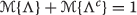for any event Λ; Axiom 3. (Subadditivity axiom) For every countable sequence of events Λ 1,Λ 2,, we haveThe tripletis called an uncertainty space. In order to obtain an uncertain measure of compound events, a product uncertain measure was defined by Liu : Axiom 4. (Product axiom) Let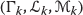be uncertainty spaces for k=1,2,. Then, the product uncertain measureis an uncertain measure satisfyingwhere Λ k are arbitrarily chosen events from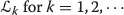, respectively.

An uncertain variable (Liu ) is a measurable function ξ from an uncertainty spaceto the set of real numbers, i.e., for any Borel set B of real numbers, the set

$$\{\xi\in B\}=\{\gamma\in\Gamma\bigm|\xi(\gamma)\in B\}$$
((1))

is an event.

In order to describe an uncertain variable in practice, the concept of uncertainty distribution was defined by Liu  as the following function,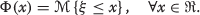((2))

The expected value of an uncertain variable ξ was defined by Liu  as an average value of the uncertain variable in the sense of uncertain measure, i.e.,((3))

provided that at least one of the two integrals is finite. Suppose the uncertain variable ξ has an uncertainty distribution Φ(x). Then, the expected value of the uncertain variable ξ is

$$E[\xi]=\int_{0}^{+\infty}(1-\Phi(r))\mathrm{d} r-\int_{-\infty}^{0} \Phi(r)\mathrm{d} r.$$
((4))

### Definition 1.

(Liu ) An uncertainty distribution Φ(x) is said to be regular if it is a continuous and strictly increasing function with respect to x at which 0<Φ(x)<1, and

$$\lim\limits_{x\rightarrow-\infty}\Phi(x)=0, \ \lim\limits_{\textit{x}\rightarrow\infty}\Phi(x)=1.$$

### Definition 2.

(Liu ) Let ξ be an uncertain variable with regular uncertainty distribution Φ. Then, the inverse function Φ −1 is called the inverse uncertainty distribution of ξ.

It has been proved that the expected value of the uncertain variable ξ with regular uncertainty distribution Φ is

$$E[\xi]={\int_{0}^{1}}\Phi^{-1}(\alpha)\mathrm{d} \alpha$$

provided that the integral exists.

Let ξ 1,ξ 2,,ξ n be independent uncertain variables with regular uncertainty distributions Φ 1, Φ 2, , Φ n , respectively. Liu  showed that if the function $$\phantom {\dot {i}\!}f(x_{1},x_{2},\cdots,x_{n})$$ is strictly increasing with respect to $$\phantom {\dot {i}\!}x_{1},x_{2},\cdots$$, x m and strictly decreasing with respect to $$\phantom {\dot {i}\!}x_{m+1},x_{m+2},\cdots,x_{n}$$, then

$$\xi=f(\xi_{1},\xi_{2},\cdots,\xi_{n})$$

is an uncertain variable with inverse uncertainty distribution

$$\Psi^{-1}(\alpha)=f\left(\Phi_{1}^{-1}(\alpha),\cdots, \Phi_{m}^{-1}(\alpha),\Phi_{m+1}^{-1}(1-\alpha),\cdots,\Phi_{n}^{-1}(1-\alpha)\right).$$
((5))

Furthermore, Liu and Ha  proved that the uncertain variable ξ=f(ξ 1,ξ 2,,ξ n ) has an expected value

$$E[\xi]={\int_{0}^{1}}f\left(\Phi_{1}^{-1}(\alpha),\cdots,\Phi_{m}^{-1}(\alpha), \Phi_{m+1}^{-1}(1-\alpha),\cdots,\Phi_{n}^{-1}(1-\alpha)\right)\mathrm{d}\alpha.$$
((6))

## Uncertain programming—basic form

Uncertain programming is a type of mathematical programming involving uncertain variables. The objectives and constraints include uncertain variables. Assume that x is a decision vector, and ξ is an uncertain vector. Assume that ξ=(ξ 1,ξ 2,,ξ n ) in which ξ 1,ξ 2,,ξ n are independent uncertain variables with regular uncertainty distributions Φ 1,Φ 2,,Φ n , respectively. Since an uncertain objective function f(x, ξ) cannot be directly minimized, we may minimize its expected value, i.e.,

$$\min_{\text{\scriptsize\boldmath \textit{x}}}E[f({\text{\boldmath \textit{x}}}, {\text{\boldmath $$\xi$$}})].$$
((7))

In addition, since the uncertain constraints g j (x, ξ)≤0(j=1,2,,p) do not define a crisp feasible set, it is naturally desired that the uncertain constraints hold with confidence levels α 1,α 2,,α p , respectively. Then, we have a set of chance constraints,((8))

In order to obtain a decision with minimum expected objective value subject to a set of chance constraints, Liu  proposed the following uncertain programming model,((9))

Without loss of generality, we assume the objective function f(x,ξ 1,ξ 2,,ξ n ) is strictly increasing with respect to $$\phantom {\dot {i}\!}\xi _{1}, \xi _{2}, \cdots, \xi _{k}$$ and strictly decreasing with respect to ξ k+1,ξ k+2,,ξ n , and the constraint functions g j (x,ξ 1,ξ 2,,ξ n ) are strictly increasing with respect to $$\xi _{1}, \xi _{2}, \cdots, \xi _{k_{j}}\phantom {\dot {i}\!}$$ and strictly decreasing with respect to $$\xi _{k_{j+1}}, \xi _{k_{j+2}}, \cdots, \xi _{n}$$ for j=1,2,,p, respectively. Then, the uncertain programming model (9) is equivalent to a crisp model,

$$\left\{\begin{array}{l}\min\limits_{\text{\scriptsize\boldmath \textit{x}}} {\int_{0}^{1}}\, f\left(\text{\boldmath \textit{x}},\Phi^{-1}_{1}(r),\cdots, \Phi^{-1}_{k}(r), \Phi^{-1}_{k+1}(1-r),\cdots,\Phi^{-1}_{n}(1-r)\right)\mathrm{d}r \\[0.2cm] \text{subject to:} \\[0.1cm] \qquad g_{j}\left(\text{\boldmath \textit{x}},\Phi^{-1}_{1}(\alpha_{j}),\cdots, \Phi^{-1}_{k_{j}}(\alpha_{j}), \Phi^{-1}_{k_{j}+1}(1-\alpha_{j}),\cdots,\Phi^{-1}_{n}(1-\alpha_{j})\right)\le 0,\\ \qquad j=1,2,\cdots,p. \end{array}\right.$$
((10))

In many cases, an uncertain programming model can be transformed to a deterministic mathematical programming model. All the methods to solve deterministic programming models can be used to solve uncertain programming models.

## Uncertain multiobjective programming

In practice, a decision maker may want to optimize two or more conflicting objectives subject to certain constraints. Uncertain multiobjective programming is a type of mathematical multiobjective programming in which the objectives and the constraints contain uncertain variables.

### Model description

Now, we consider an uncertain multiobjective programming model. Assume that x is a decision vector, ξ is an uncertain vector, and f 1(x, ξ),f 2(x, ξ),,f m (x, ξ) are return functions. Since the objective functions are uncertain variables, they cannot be directly maximized. Instead, we maximize their expected values, i.e.,

$$E[f_{1}(\text{\boldmath \textit{x}},{\text{\boldmath $$\xi$$}})],E[f_{2}(\text{\boldmath \textit{x}},{\text{\boldmath $$\xi$$}})],\cdots,E[f_{m}(\text{\boldmath \textit{x}},{\text{\boldmath $$\xi$$}})].$$

Assume that the constraints are

$$g_{i}(\text{\boldmath \textit{x}}, {\text{\boldmath $$\xi$$}}) \le 0,\ i=1,2,\cdots,p.$$
((11))

Since g i (x, ξ) are uncertain variables, the inequalities (11) do not define a crisp feasible set. Instead, we hope that the inequalities (11) hold with given confidence levels α i . Then, the feasible set is defined by the chance constraints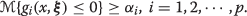Thus, we have the following uncertain multiobjective programming model,((12))

### Definition 3.

A solution x is said to be feasible to the uncertain multiobjective programming (12) if((13))

for j=1,2,,p.

### Definition 4.

A feasible solution x is said to be a Pareto solution to the uncertain multiobjective programming (12) if there is no feasible solution x such that

$$E[f_{i}(\text{\boldmath \textit{x}},{\text{\boldmath $$\xi$$}})]\ge E[f_{i}(\text{\boldmath \textit{x}}^{*},{\text{\boldmath $$\xi$$}})],\quad i=1,2,\cdots,m$$
((14))

and E[f j (x, ξ)]>E[f j (x , ξ)] for at least one index j.

### Theorem 1.

Assume f(x, ξ)=h 1(x)ξ 1+h 2(x)ξ 2++h n (x)ξ n +h 0(x) where h 1(x),h 2(x),,h n (x), h 0(x) are real-valued functions and ξ 1,ξ 2,,ξ n are independent uncertain variables. Then,

$$E[f(\text{\boldmath \textit{x}},{\text{\boldmath $$\xi$$}})]=h_{1}(\text{\boldmath \textit{x}})E[\xi_{1}]+h_{2}(\text{\boldmath \textit{x}})E[\xi_{2}]+\cdots+h_{n}(\text{\boldmath \textit{x}})E[\xi_{n}]+h_{0}(\text{\boldmath \textit{x}}).$$
((15))

### Proof.

Since ξ 1,ξ 2,,ξ n are independent uncertain variables, it follows from the linearity of expected value operator of uncertain variables that

$$E[f(\text{\boldmath \textit{x}},{\text{\boldmath $$\xi$$}})]=h_{1}(\text{\boldmath \textit{x}})E[\xi_{1}]+h_{2}(\text{\boldmath \textit{x}})E[\xi_{2}]+\cdots+h_{n}(\text{\boldmath \textit{x}})E[\xi_{n}]+h_{0}(\text{\boldmath \textit{x}}).$$

The theorem is proved.

### Theorem 2.

Assume that ξ 1,ξ 2,,ξ n are independent uncertain variables and h 1(x),h 2(x),,h n (x), h 0(x) are real-valued functions. Then,

$$E[h_{1}(\text{\boldmath \textit{x}})\xi_{1}+h_{2}(\text{\boldmath \textit{x}})\xi_{2}+\cdots+h_{n}(\text{\boldmath \textit{x}})\xi_{n}+h_{0}(\text{\boldmath \textit{x}})]\le 0$$
((16))

holds if and only if

$$h_{1}(\text{\boldmath \textit{x}})E[\xi_{1}]+h_{2}(\text{\boldmath \textit{x}})E[\xi_{2}]+\cdots+h_{n}(\text{\boldmath \textit{x}})E[\xi_{n}]+h_{0}(\text{\boldmath \textit{x}})\le 0.$$
((17))

### Proof.

Since ξ 1,ξ 2,,ξ n are independent uncertain variables, it follows from Theorem 1 that

\begin{aligned} & E[h_{1}(\text{\boldmath \textit{x}})\xi_{1}+h_{2}(\text{\boldmath \textit{x}})\xi_{2}+\cdots+h_{n}(\text{\boldmath \textit{x}})\xi_{n}+h_{0}(\text{\boldmath \textit{x}})]=h_{1}(\text{\boldmath \textit{x}})E[\xi_{1}]+h_{2}(\text{\boldmath \textit{x}})\\&E[\xi_{2}]+\cdots+h_{n}(\text{\boldmath \textit{x}})E[\xi_{n}]+h_{0}(\text{\boldmath \textit{x}}). \end{aligned}
((18))

Thus, two sides of (18) will satisfy the same inequality. The theorem is proved.

### Theorem 3.

Let ξ 1,ξ 2,,ξ n be independent uncertain variables with regular uncertainty distributions Φ 1,Φ 2, ,Φ n , respectively. Assume g j are strictly increasing with respect to $$\xi _{1},\xi _{2},\cdots,\xi _{s_{j}}\phantom {\dot {i}\!}$$ and strictly decreasing with respect to $$\xi _{s_{j}+1}, \xi _{s_{j}+2}, \cdots, \xi _{n}$$, j=1,2,,m, respectively. Then,are equivalent to

$$g_{j}\left(\text{\boldmath \textit{x}}, \Phi^{-1}_{1}(\alpha_{j}), \Phi^{-1}_{2}(\alpha_{j}), \cdots,\Phi^{-1}_{s_{j}}(\alpha_{j}),\Phi^{-1}_{s_{j}+1}(1-\alpha_{j}), \cdots, \Phi^{-1}_{n}(1-\alpha_{j})\right) \le 0,$$

j=1,2,,p, respectively.

### Proof.

Since the constraint functions g j are strictly increasing with respect to $${\xi }_{1},{\xi }_{2},\cdots,{\xi }_{{s}_{j}}\phantom {\dot {i}\!}$$ and strictly decreasing with respect to $$\xi _{s_{j}+1}, \xi _{s_{j}+2}, \cdots, \xi _{n}$$, for j=1,2,,m, respectively, it follows from the operational law of uncertain variables that the inverse uncertainty distributions of the uncertain variables g j (x,ξ) are

$$\Psi^{-1}_{j}(\alpha_{j})=g_{j}\left({x}, \Phi^{-1}_{1}(\alpha_{j}), \Phi^{-1}_{2}(\alpha_{j}), \cdots,\Phi^{-1}_{s_{j}}(\alpha_{j}),\Phi^{-1}_{s_{j+1}}(1-\alpha_{j}), \cdots, \Phi^{-1}_{n}(1-\alpha_{j})\right),$$

j=1,2,,p, respectively. According to the the definition of inverse uncertainty distribution, the inequalities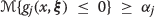and $$\Psi _{j}^{-1}(\alpha _{j})\le 0$$ are equivalent, for j=1,2,,p, respectively. Thus, the theorem is proved.

### Equivalent crisp model

From the mathematical viewpoint, there is no difference between deterministic mathematical programming and uncertain programming except for the fact that there exist uncertain variables in the latter. In fact, the uncertain multiobjective programming model (12) is equivalent to a deterministic multiobjective programming model. Without loss of generality, we assume that f i are strictly increasing with respect to $$\xi _{1}, \xi _{2},\cdots, \xi _{k_{i}}\phantom {\dot {i}\!}$$ and strictly decreasing with respect to $$\xi _{k_{i}+1}, \xi _{k_{i}+2}, \cdots, \xi _{n},\ i=1,2,\cdots,m$$, respectively. Then, we have

$$E[f_{i}({\text{\boldmath \textit{x}}},\xi_{1},\xi_{2},\cdots,\xi_{n})]={\int_{0}^{1}} \,f_{i}\left({\text{\boldmath \textit{x}}},\Phi^{-1}_{1}(r),\cdots, \Phi^{-1}_{k_{i}}(r), \Phi^{-1}_{k_{i}+1}(1-r),\cdots,\Phi^{-1}_{n}(1-r)\right)\mathrm{d} r,$$

for i=1,2,,m. It follows from Theorem 3 that the uncertain multiobjective programming model (12) is equivalent to

$$\left\{\begin{array}{l} \max\limits_{\text{\scriptsize\boldmath \textit{x}}} \left[{\int_{0}^{1}} \,f_{i}(\text{\boldmath \textit{x}},\Phi^{-1}_{1}(r),\cdots, \Phi^{-1}_{k}(r), \Phi^{-1}_{k+1}(1-r),\cdots,\Phi^{-1}_{n}(1-r))\mathrm{d} r, i=1,2,\cdots,m.\right] \\[0.2cm] \text{subject to:} \\[0.1cm] \qquad g_{j}(\text{\boldmath \textit{x}},\Phi^{-1}_{1}(\alpha_{j}),\cdots, \Phi^{-1}_{k_{j}}(\alpha_{j}), \Phi^{-1}_{k_{j}+1}(1-\alpha_{j}),\cdots,\Phi^{-1}_{n}(1-\alpha_{j}))\le 0,\\\qquad j=1,2,\cdots,p. \end{array}\right.$$
((19))

The common method to solve multiobjective programming model is to translate it to a single objective programming model. If the decision maker already has weights ω i for objectives i with $$\sum \limits _{i=1}^{m}\omega _{i}=1$$, then the multiobjective programming model becomes

$$\left\{\begin{array}{l} \max\limits_{\text{\scriptsize\boldmath \textit{x}}}\sum\limits_{i=1}^{m}\omega_{i}{\int_{0}^{1}} \,f_{i}\left(\text{\boldmath \textit{x}},\Phi^{-1}_{1}(r),\cdots, \Phi^{-1}_{k}(r), \Phi^{-1}_{k+1}(1-r),\cdots,\Phi^{-1}_{n}(1-r)\right)\mathrm{d} r \\[0.2cm] \text{subject to:} \\[0.1cm] \qquad g_{j}\left(\text{\boldmath \textit{x}},\Phi^{-1}_{1}(\alpha_{j}),\cdots, \Phi^{-1}_{k_{j}}(\alpha_{j}), \Phi^{-1}_{k_{j}+1}(1-\alpha_{j}),\cdots,\Phi^{-1}_{n}(1-\alpha_{j})\right)\le 0,\\\qquad j=1,2,\cdots,p. \end{array}\right.$$
((20))

## Uncertain goal programming

In order to balance multiple conflicting objectives, a decision maker may establish a hierarchy of importance among these incompatible goals so as to satisfy as many goals as possible in the specified order. Thus, we have an uncertain goal programming,((21))

where P j are the preemptive priority factors which express the relative importance of various goals, P j P j+1, for all j, u ij are the weighting factors corresponding to positive deviation for goals i with priority j assigned, v ij are the weighting factors corresponding to negative deviation for goals i with priority j assigned, $$d^{+}_{i}$$ are the positive deviations from the target of goals i, $$d_{i}^{-}$$ are the negative deviations from the target of goals i, f i are objective functions in goal constraints, g j are functions in real constraints, b i are the target values, l is the number of priorities, m is the number of goal constraints, and p is the number of real constraints.

### Equivalent crisp model

In fact, the uncertain goal programming model (21) is equivalent to a deterministic goal programming model. Let ξ 1,ξ 2,,ξ n be independent uncertain variables with regular uncertainty distributions Φ 1,Φ 2,,Φ n , respectively. Without loss of generality, we assume that f i are strictly increasing with respect to $$\xi _{1}, \xi _{2}, \cdots, \xi _{k_{i}}\phantom {\dot {i}\!}$$ and strictly decreasing with respect to $$\xi _{k_{i}+1}, \xi _{k_{i}+2}, \cdots, \xi _{n},$$ for i=1,2,,m, respectively. Then, we have

$$E[f_{i}(x,\xi_{1},\xi_{2},\cdots,\xi_{n})]={\int_{0}^{1}} f_{i}\left(x,\Phi^{-1}_{1}(r),\cdots, \Phi^{-1}_{k_{i}}(r), \Phi^{-1}_{k_{i}+1}(1-r),\cdots,\Phi^{-1}_{n}(1-r)\right)\mathrm{d} r,$$

for i=1,2,,m. Assume g j are strictly increasing with respect to $$\xi _{1}, \xi _{2}, \cdots, \xi _{s_{j}}\phantom {\dot {i}\!}$$ and strictly decreasing with respect to $$\xi _{s_{j}+1}, \xi _{s_{j}+2}, \cdots, \xi _{n}$$, for j=1,2,,p, respectively. Then,are equivalent to

$$g_{j}(x, \Phi^{-1}_{1}(\alpha_{j}), \Phi^{-1}_{2}(\alpha_{j}), \cdots,\Phi^{-1}_{s_{j}}(\alpha_{j}),\Phi^{-1}_{s_{j}+1}(\alpha_{j})\cdots, \Phi^{-1}_{n}(1-\alpha_{j})) \le 0,$$

for j=1,2,,p, respectively. Thus, the uncertain goal programming model (21) is equivalent to

{} {\fontsize{8.6pt}{9.6pt}\selectfont{\begin{aligned} \left\{\!\!\begin{array}{l} \min\sum\limits^{l}_{j=1}P_{j}\sum\limits^{m}_{i=1}\left(u_{ij}d^{+}_{i}+v_{ij}d^{-}_{i}\right)\\ [0.4 cm] \text{subject to:} \\ [0.1 cm] \begin{array}{ll} \quad{\int_{0}^{1}}\,f_{i}\left(\text{\boldmath \textit{x}},\Phi^{-1}_{1}(r),\cdots, \Phi^{-1}_{k_{i}}(r), \Phi^{-1}_{k_{i}+1}(1-r),\cdots,\Phi^{-1}_{n}(1-r)\right)\mathrm{d} r+d^{-}_{i}-d^{+}_{i}=b_{i},&i=1,2,\cdots,m\\[0.1 cm] \quad g_{j}\left(\text{\boldmath \textit{x}},\Phi^{-1}_{1}(\alpha_{j}),\cdots, \Phi^{-1}_{s_{j}}(\alpha_{j}), \Phi^{-1}_{s_{j}+1}(1-\alpha_{j}),\cdots,\Phi^{-1}_{n}(1-\alpha_{j})\right)\le 0,&j=1,2,\cdots,p\\[0.1cm] \quad d_{i}^{+},d_{i}^{-}\ge 0,&i=1,2,\cdots,m. \end{array}\end{array}\right. \end{aligned}}}

## Conclusions

This paper provided an uncertain multiobjective programming model and an uncertain goal programming model which are mathematical programming models involving uncertain variables. These models were turned to a crisp multiobjective programming model and a crisp goal programming model using operational law of uncertain variables via inverse uncertainty distributions, respectively. Several properties were also investigated in this paper.

## References

1. Charnes, A, Cooper, WW: Chance-constrained programming. Manag. Sci. 6(1), 73–79 (1959).

2. Charnes, A, Copper, WW: Management models and industrial applications of linear programming. Wiley, New York (1961).

3. Dantzig, GB: Linear programming under uncertainty. Manag. Sci. 1, 197–206 (1955).

4. Gao, Y: Uncertain inference control for balancing inverted pendulum. Fuzzy Optim. Decis. Making. 11(4), 481–492 (2012).

5. Kahneman, D, Tversky, A: Prospect theory: an analysis of decision under risk. Econometrica. 47(2), 263–292 (1979).

6. Koopmans, TC: Analysis of production as an efficient combination of activities. Wiley, New York (1951).

7. Liu, B: Theory and practice of uncertain programming. 2nd ed. Springer-Verlag, Berlins (2009).

8. Liu, B: Uncertainty theory. 2nd ed. Springer-Verlag, Berlin (2007).

9. Liu, B: Fuzzy process, hybrid process and uncertain process. J. Uncertain Syst. 2(1), 3–16 (2008).

10. Liu, B: Some research problems in uncertainty theory. J. Uncertain Syst. 31, 3–10 (2009).

11. Liu, B: Uncertain set theory and uncertain inference rule with application to uncertain control. J. Uncertain Syst. 4(2), 83–98 (2010).

12. Liu, B: Uncertain risk analysis and uncertain reliability analysis. J. Uncertain Syst. 4(3), 163–170 (2010).

13. Liu, B: Uncertainty theory: a branch of mathematics for modeling human uncertainty. Springer-Verlag, Berlin (2010).

14. Liu, B: Why is there a need for uncertainty theory. J. Uncertain Syst. 6(1), 3–10 (2012).

15. Liu, B: Uncertainty theory. 4th ed. Springer-Verlag, Berlin (2015).

16. Liu, B, Yao, K: Uncertain multilevel programming: algorithm and applications. Comput. Ind. Eng (2015). doi:http://dx.doi.org/10.1016/j.cie.2014.09.029.

17. Liu, YH, Ha, MH: Expected value of function of uncertain variables. J. Uncertain Syst. 4(3), 181–186 (2010).

18. Miettinen, K: Nonlinear multiobjective optimization. IEEE Trans. Syst. Man Cybern. Syst. Hum. 38(2), 319–330 (2008).

19. Von Neumann, J, Morgenstern, O: Theory of games and economic behavior, 1st ed.Princeton University Press, Princeton (1944).

20. Peng, ZX, Chen, XW: Uncertain systems are universal approximators. J. Uncertain. Anal. Appl. 2, Article, 13 (2014).

21. Wang, XS, Peng, ZX: Method of moments for estimating uncertainty distributions. J. Uncertain. Anal. Appl. 2, Article, 5 (2014).

## Acknowledgements

This work was supported by National Natural Science Foundation of China Grant No.61273044 and No. 61304182.

## Author information

Authors

### Corresponding author

Correspondence to Xiaowei Chen.

## Rights and permissions